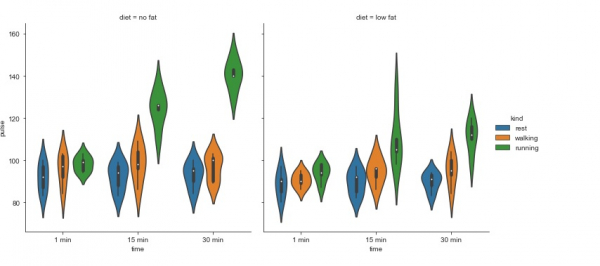# How can FacetGrid be used to visualize data in Python Seaborn Library?

The barplot function establishes the relationship between a categorical variable and a continuous variable. Data is represented in the form of rectangular bars where the length of the bar indicates the proportion of data in that specific category.

Point plots are similar to bar plots but instead of representing the fill bar, the estimated value of the data point is represented by a point at a specific height on the other axis.

Categorical data can be visualized using categorical scatter plots or two separate plots with the help of pointplot or a higher level function known as factorplot. The factorplot function draws a categorical plot on a FacetGrid, with the help of parameter ‘kind’.

The value to ‘kind’ parameter here would be ‘violin’. FacetGrid uses ‘pointplot’ function by default.

Let us understand how a factorplot can be used to create a violin graph.

FacetGrid is a matrix of panels, which is defined as row and column by dividing all the variables. Because of the panels, a single plot looks like a multitude of plots.

Facet grid is useful in analysing all the different types of combinations of two discrete variables. Here’s the example −

## Example

import pandas as pd
import seaborn as sb
from matplotlib import pyplot as plt
sb.factorplot(x = "time", y = "pulse", hue = "kind", kind = 'violin', col = "diet", data = my_df);
plt.show()

## Output## Explanation

• The required packages are imported.
• The input data is ‘exercise’ which is loaded from the seaborn library.
• This data is stored in a dataframe.
• This data is visualized using the ‘factorplot’ function.
• Here, the dataframe is supplied as parameter.
• The ‘kind’ parameter is specified as ‘violin’ here.
• The ‘hue’ and the ‘col’ parameters are also specified.
• Also, the x and y values are specified.
• This data is displayed on the console.

Updated on: 11-Dec-2020

117 Views